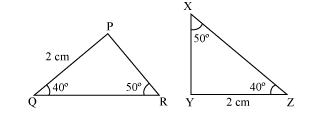# How the AAS congruence criteria works?

ASA stands for “Angle, Side, Angle”:

If two angles and the included side of one triangle are equal to two angles and the included side of another triangle, then the triangles are congruent.In $\Delta P Q R$ and $\Delta X Y Z$ $\angle Q=\angle Z$

$\angle \mathrm{R}=\angle \mathrm{X}$

$\mathrm{PQ}=\mathrm{YZ}$ Non-included side between the two angles.

So,by AAS congruence rule,

$\Delta P Q R \equiv \triangle X Y Z$

Updated on: 10-Oct-2022

27 Views